• 用法：DataFrame.drop(labels=None,axis=0, index=None, columns=None, inplace=False) ...inplace=False，默认该删除操作不改变原数据，而是返回一个执行删除操作后的新dataframe； inplace=True，则会直接在原...
用法：DataFrame.drop(labels=None,axis=0, index=None, columns=None, inplace=False)
参数说明： labels 就是要删除的行列的名字，用列表给定 axis 默认为0，指删除行，因此删除columns时要指定axis=1； index 直接指定要删除的行 columns 直接指定要删除的列 inplace=False，默认该删除操作不改变原数据，而是返回一个执行删除操作后的新dataframe； inplace=True，则会直接在原数据上进行删除操作，删除后无法返回。
因此，删除行列有两种方式： 1）labels=None,axis=0 的组合 2）index或columns直接指定要删除的行或列
例子：
>>>df = pd.DataFrame(np.arange(12).reshape(3,4), columns=['A', 'B', 'C', 'D'])

>>>df

A   B   C   D

0  0   1   2   3

1  4   5   6   7

2  8   9  10  11

#Drop columns,两种方法等价

>>>df.drop(['B', 'C'], axis=1)

A   D

0  0   3

1  4   7

2  8  11

>>>df.drop(columns=['B', 'C'])

A   D

0  0   3

1  4   7

2  8  11

# 第一种方法下删除column一定要指定axis=1,否则会报错
>>> df.drop(['B', 'C'])

ValueError: labels ['B' 'C'] not contained in axis

#Drop rows
>>>df.drop([0, 1])

A  B   C   D

2  8  9  10  11

>>> df.drop(index=[0, 1])

A  B   C   D

2  8  9  10  11

Life is short, You need Python~
展开全文dataframe drop
• pandas中，dropna函数分别存在于DataFrame、Series和Index中，下面我们以DataFrame.dropna函数为例进行介绍，Series和Index中的参数意义同DataFrame...DataFrame.dropna(axis=0, how=‘any’, thresh=None, subset=No
在pandas中，dropna函数分别存在于DataFrame、Series和Index中，下面我们以DataFrame.dropna函数为例进行介绍，Series和Index中的参数意义同DataFrame中大致相同。
pandas.DataFrame.dropna函数
函数参数
DataFrame.dropna(axis=0, how=‘any’, thresh=None, subset=None, inplace=False)
参数名称参数取值参数意义axis0 or ‘index’, 1 or ‘columns’ , default 0确定是删除包含缺失值的行还是列how‘any’ or ‘all’, default ‘any’表明是至少存在一个NAN值还是全为NAN值时执行删除操作threshint, 可选指定存在多少个NAN值才进行删除操作subsetarray, 可选可选子集列表inplacebool, default False如果为真，执行inplace操作，并返回None
import pandas as pd
import numpy as np

# 定义一个DataFrame
df = pd.DataFrame({"name": ['张三', '李四', '王五'],
"born": [pd.NaT, pd.Timestamp("1998-04-25"),
pd.NaT]})


运行结果如下：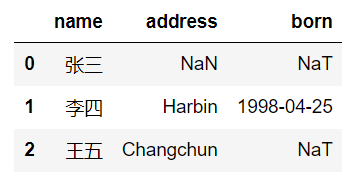1.使用默认参数进行操作
df.dropna()

运行结果如下：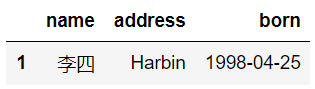由运行结果看出，该函数把所有含有NAN值的行都执行了删除操作。
2.修改axis参数
df.dropna(axis=1)              # 删除列
df.dropna(axis='columns')      # 删除列

运行结果如下：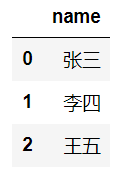由结果可以看出，通过改变axis参数，可以控制删除操作执行的维度，0代表删除行，1代表删除列。
3.修改how参数
df.dropna(how='all')

运行结果如下：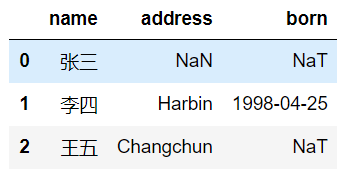有运行结果可以看出，数据并没有发生变化，这是因为在该数据中，并没有存在整行全部为NAN值的情况，所以不会进行删除操作。
4.修改thresh参数
df.dropna(thresh=2)

运行结果如下：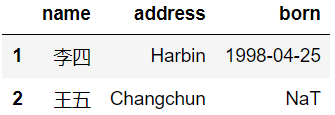通过运行结果可以看出，当thresh=2时，说明将所有NAN值个数大于等于2的行或者列进行删除操作。
5.修改subset参数
df.dropna(subset=['name', 'address'])

运行结果如下：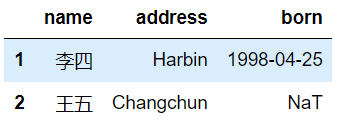通过结果截图可以看出，通过subset参数，可以选定一个数据的子集，例如代码中的[‘name’, ‘address’]，在这个子集上进行删除操作，而不考虑born中的数据是否含有NAN值。
6.修改inplace参数
import pandas as pd

df = pd.DataFrame({"name": ['Alfred', 'Batman', 'Catwoman'],
"toy": [np.nan, 'Batmobile', 'Bullwhip'],
"born": [pd.NaT, pd.Timestamp("1940-04-25"),
pd.NaT]})

data = df.dropna(inplace=True)
print('----- the df is ------')
print(df)
print('----- the data is ------')
print(data)

运行结果如下：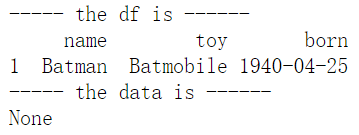由运行结果可以得出： inplace=True，表示不创建新对象，直接在原始对象上进行修改，返回None； inplace=False，表示创建新对象，返回创建对象的修改结果。
参考文献
DataFrame.dropna官方文档 Series.dropna官方文档 Index.dropna官方文档
展开全文python
• ## pandas用法总结

万次阅读 多人点赞 2018-06-07 10:49:03
1、首先导入pandas库，一般都会用到numpy库，所以我们先导入备用： import numpy as np import pandas as pd 2、导入CSV或者xlsx文件： df = pd.DataFrame(pd.read_csv(‘name.csv’,header=1)) df = pd....
一、生成数据表
各位读者朋友们，由于更新blog不易，如果觉得这篇blog对你有用的话，麻烦关注，点赞，收藏一下哈，十分感谢。
1、首先导入pandas库，一般都会用到numpy库，所以我们先导入备用：
import numpy as np
import pandas as pd

2、导入CSV或者xlsx文件：
df = pd.DataFrame(pd.read_csv('name.csv',header=1))

或者
import pandas as pd
from collections import namedtuple

items = []

with codecs.open('reply.pv.07', 'r', 'utf-8') as f:
for line in f:
line_split = line.strip().split('\t')
items.append(Item(line_split.strip(), line_split.strip()))


3、用pandas创建数据表：
df = pd.DataFrame({"id":[1001,1002,1003,1004,1005,1006],
"date":pd.date_range('20130102', periods=6),
"city":['Beijing ', 'SH', ' guangzhou ', 'Shenzhen', 'shanghai', 'BEIJING '],
"age":[23,44,54,32,34,32],
"category":['100-A','100-B','110-A','110-C','210-A','130-F'],
"price":[1200,np.nan,2133,5433,np.nan,4432]},
columns =['id','date','city','category','age','price'])

二、数据表信息查看
1、维度查看：
df.shape

2、数据表基本信息（维度、列名称、数据格式、所占空间等）：
df.info()

3、每一列数据的格式：
df.dtypes

4、某一列格式：
df['B'].dtype

5、空值：
df.isnull()

6、查看某一列空值：
df['B'].isnull()

7、查看某一列的唯一值：
df['B'].unique()

8、查看数据表的值：
df.values

9、查看列名称：
df.columns

10、查看前5行数据、后5行数据：
df.head() #默认前5行数据
df.tail()    #默认后5行数据

三、数据表清洗
1、用数字0填充空值：
df.fillna(value=0)

2、使用列prince的均值对NA进行填充：
df['prince'].fillna(df['prince'].mean())

3、清楚city字段的字符空格：
df['city']=df['city'].map(str.strip)

4、大小写转换：
df['city']=df['city'].str.lower()

5、更改数据格式：
df['price'].astype('int')

6、更改列名称：
df.rename(columns={'category': 'category-size'})

7、删除后出现的重复值：
df['city'].drop_duplicates()

8 、删除先出现的重复值：
df['city'].drop_duplicates(keep='last')

9、数据替换：
df['city'].replace('sh', 'shanghai')

四、数据预处理
df1=pd.DataFrame({"id":[1001,1002,1003,1004,1005,1006,1007,1008],
"gender":['male','female','male','female','male','female','male','female'],
"pay":['Y','N','Y','Y','N','Y','N','Y',],
"m-point":[10,12,20,40,40,40,30,20]})

1、数据表合并
1.1 merge
df_inner=pd.merge(df,df1,how='inner')  # 匹配合并，交集
df_left=pd.merge(df,df1,how='left')        #
df_right=pd.merge(df,df1,how='right')
df_outer=pd.merge(df,df1,how='outer')  #并集

1.2 append
result = df1.append(df2)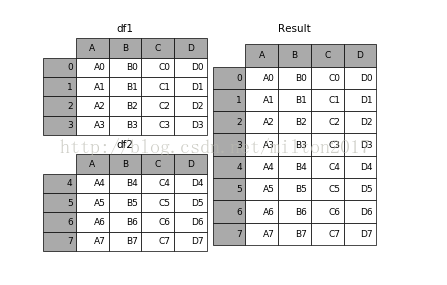1.3 join
result = left.join(right, on='key')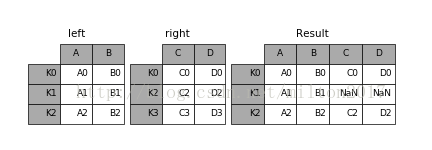1.4 concat
pd.concat(objs, axis=0, join='outer', join_axes=None, ignore_index=False,
keys=None, levels=None, names=None, verify_integrity=False,
copy=True)

objs︰ 一个序列或系列、 综合或面板对象的映射。如果字典中传递，将作为键参数，使用排序的键，除非它传递，在这种情况下的值将会选择 （见下文）。任何没有任何反对将默默地被丢弃，除非他们都没有在这种情况下将引发 ValueError。axis: {0，1，…}，默认值为 0。要连接沿轴。join: {‘内部’、 ‘外’}，默认 ‘外’。如何处理其他 axis(es) 上的索引。联盟内、 外的交叉口。ignore_index︰ 布尔值、 默认 False。如果为 True，则不要串联轴上使用的索引值。由此产生的轴将标记 0，…，n-1。这是有用的如果你串联串联轴没有有意义的索引信息的对象。请注意在联接中仍然受到尊重的其他轴上的索引值。join_axes︰ 索引对象的列表。具体的指标，用于其他 n-1 轴而不是执行内部/外部设置逻辑。 keys︰ 序列，默认为无。构建分层索引使用通过的键作为最外面的级别。如果多个级别获得通过，应包含元组。levels︰ 列表的序列，默认为无。具体水平 （唯一值） 用于构建多重。否则，他们将推断钥匙。names︰ 列表中，默认为无。由此产生的分层索引中的级的名称。verify_integrity︰ 布尔值、 默认 False。检查是否新的串联的轴包含重复项。这可以是相对于实际数据串联非常昂贵。副本︰ 布尔值、 默认 True。如果为 False，请不要，不必要地复制数据。
例子：1.frames = [df1, df2, df3]
2.result = pd.concat(frames)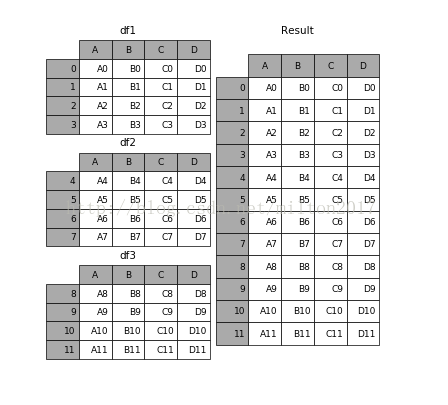2、设置索引列
df_inner.set_index('id')

3、按照特定列的值排序：
df_inner.sort_values(by=['age'])

4、按照索引列排序：
df_inner.sort_index()

5、如果prince列的值>3000，group列显示high，否则显示low：
df_inner['group'] = np.where(df_inner['price'] > 3000,'high','low')

6、对复合多个条件的数据进行分组标记
df_inner.loc[(df_inner['city'] == 'beijing') & (df_inner['price'] >= 4000), 'sign']=1

7、对category字段的值依次进行分列，并创建数据表，索引值为df_inner的索引列，列名称为category和size
pd.DataFrame((x.split('-') for x in df_inner['category']),index=df_inner.index,columns=['category','size']))

8、将完成分裂后的数据表和原df_inner数据表进行匹配
df_inner=pd.merge(df_inner,split,right_index=True, left_index=True)

五、数据提取
主要用到的三个函数：loc,iloc和ix，loc函数按标签值进行提取，iloc按位置进行提取，ix可以同时按标签和位置进行提取。
1、按索引提取单行的数值
df_inner.loc

2、按索引提取区域行数值
df_inner.iloc[0:5]

3、重设索引
df_inner.reset_index()

4、设置日期为索引
df_inner=df_inner.set_index('date')

5、提取4日之前的所有数据
df_inner[:'2013-01-04']

6、使用iloc按位置区域提取数据
df_inner.iloc[:3,:2] #冒号前后的数字不再是索引的标签名称，而是数据所在的位置，从0开始，前三行，前两列。

7、适应iloc按位置单独提起数据
df_inner.iloc[[0,2,5],[4,5]] #提取第0、2、5行，4、5列

8、使用ix按索引标签和位置混合提取数据
df_inner.ix[:'2013-01-03',:4] #2013-01-03号之前，前四列数据

9、判断city列的值是否为北京
df_inner['city'].isin(['beijing'])

10、判断city列里是否包含beijing和shanghai，然后将符合条件的数据提取出来
df_inner.loc[df_inner['city'].isin(['beijing','shanghai'])]

11、提取前三个字符，并生成数据表
pd.DataFrame(category.str[:3])

六、数据筛选
使用与、或、非三个条件配合大于、小于、等于对数据进行筛选，并进行计数和求和。
1、使用“与”进行筛选
df_inner.loc[(df_inner['age'] > 25) & (df_inner['city'] == 'beijing'), ['id','city','age','category','gender']]

2、使用“或”进行筛选
df_inner.loc[(df_inner['age'] > 25) | (df_inner['city'] == 'beijing'), ['id','city','age','category','gender']].sort(['age'])

3、使用“非”条件进行筛选
df_inner.loc[(df_inner['city'] != 'beijing'), ['id','city','age','category','gender']].sort(['id'])

4、对筛选后的数据按city列进行计数
df_inner.loc[(df_inner['city'] != 'beijing'), ['id','city','age','category','gender']].sort(['id']).city.count()

5、使用query函数进行筛选
df_inner.query('city == ["beijing", "shanghai"]')

6、对筛选后的结果按prince进行求和
df_inner.query('city == ["beijing", "shanghai"]').price.sum()

七、数据汇总
主要函数是groupby和pivote_table
1、对所有的列进行计数汇总
df_inner.groupby('city').count()

2、按城市对id字段进行计数
df_inner.groupby('city')['id'].count()

3、对两个字段进行汇总计数
df_inner.groupby(['city','size'])['id'].count()

4、对city字段进行汇总，并分别计算prince的合计和均值
df_inner.groupby('city')['price'].agg([len,np.sum, np.mean])

八、数据统计
数据采样，计算标准差，协方差和相关系数
1、简单的数据采样
df_inner.sample(n=3)

2、手动设置采样权重
weights = [0, 0, 0, 0, 0.5, 0.5]
df_inner.sample(n=2, weights=weights)

3、采样后不放回
df_inner.sample(n=6, replace=False)

4、采样后放回
df_inner.sample(n=6, replace=True)

5、 数据表描述性统计
df_inner.describe().round(2).T #round函数设置显示小数位，T表示转置

6、计算列的标准差
df_inner['price'].std()

7、计算两个字段间的协方差
df_inner['price'].cov(df_inner['m-point'])

8、数据表中所有字段间的协方差
df_inner.cov()

9、两个字段的相关性分析
df_inner['price'].corr(df_inner['m-point']) #相关系数在-1到1之间，接近1为正相关，接近-1为负相关，0为不相关

10、数据表的相关性分析
df_inner.corr()

九、数据输出
分析后的数据可以输出为xlsx格式和csv格式
1、写入Excel
df_inner.to_excel('excel_to_python.xlsx', sheet_name='bluewhale_cc')

2、写入到CSV
df_inner.to_csv('excel_to_python.csv')

展开全文python 数据处理
• 不得不说DataFrame现在很火，已经有很多库都是基于DataFrame写的，而且它用起来也很方便，读excel只需要一行代码，起使用xlrd的日子，至今还脑壳疼，所以对于一个用python做数据处理的人来说，pandas是必须要了解的...
不得不说DataFrame现在很火，已经有很多库都是基于DataFrame写的，而且它用起来也很方便，读excel只需要一行代码，想起使用xlrd的日子，至今还脑壳疼，所以对于一个用python做数据处理的人来说，pandas是必须要了解的。对于一个数据处理工具来说，读写是最基本的，下面是我最近整理的关于pandas一些基本本操作，主要包括以下内容：
如何创建DataFrame如何读取DataFrame的值，读一行/列、读多行/列如何对DataFrame赋值如何对DataFrame插入一（多）行/列如何删除DataFrame的一（多）行/列

开始前先引入两个库
import pandas as pd
import numpy as np

1 创建DataFrame
1.1 利用字典创建
data={"one":np.random.randn(4),"two":np.linspace(1,4,4),"three":['zhangsan','李四',999,0.1]}
df=pd.DataFrame(data,index=[1,2,3,4])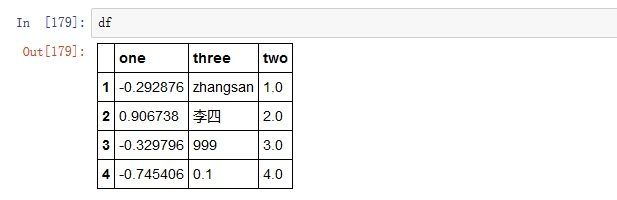如果创建df时不指定索引，默认索引将是从0开时，步长为1的数组。df的行、列可以是不同的数据类型，同行也可以有多种数据类型。df创建完成后可以重新设置索引，通常用到3个函数：set_index、reset_index、reindex。
set _index用于将df中的一行或多行设置为索引。 df.set_index(['one'],drop=False) ordf.set_index('one) df.set_index(['one','two']) 参数drop默认为True，意为将该列设置为索引后从数据中删除，如果设为False，将继续在数据中保留该行。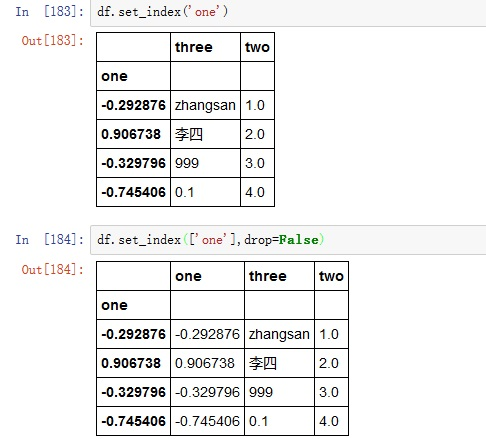如果要设置的索引不在数据中，可以通过 df.index=['a','b','c','d']reset_index用于将索引还原成默认值，即从0开始步长为1的数组。 df.reset_index(drop=True) 参数drop默认值为False，意为将原来的索引做为数据列保留，如果设为True，原来的索引会直接删除。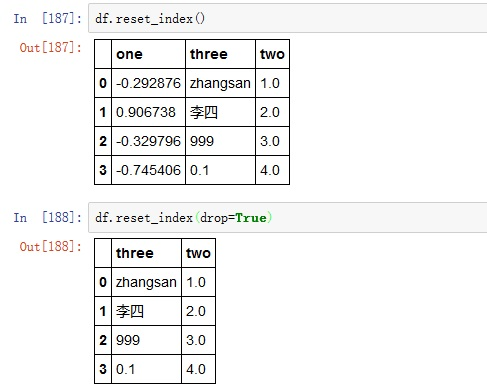reindex比较复杂，也不常用到，这里是基础篇，不做大量说明，感兴趣的朋友可以看官方文档
1.2 利用数组创建
data=np.random.randn(6,4)#创建一个6行4列的数组
df=pd.DataFrame(data,columns=list('ABCD'),index=[1,2,'a','b','2006-10-1','第六行'])

1.3 创建一个空DataFrame
pd.DataFrame(columns=('id','name','grade','class'))

为了便于理解，以下面DataFrame为例，对其读写操作展开说明：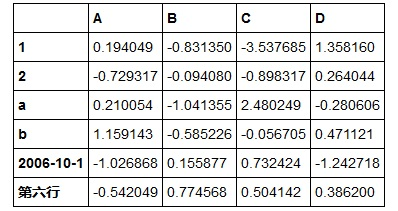2 读DataFrame
2.1按列读取
方法1：df.列名 该方法每次只能读取一列。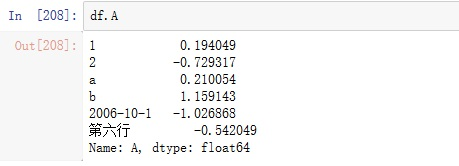方法2：df['列名']or df[['列名']]、df[['列名1','列名2','列名n']]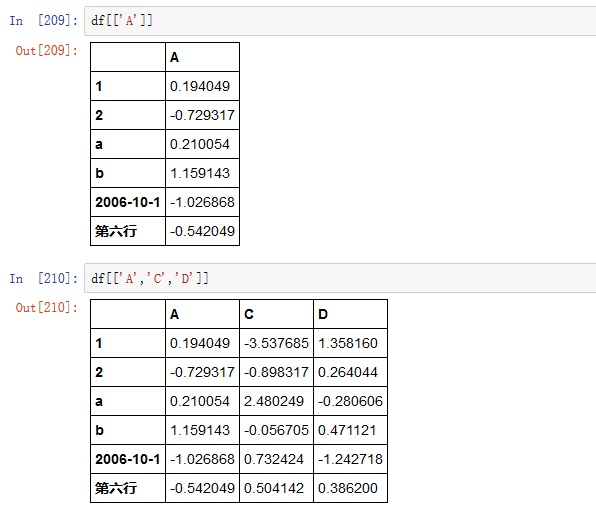ps：df['A']和 df[['A']]都能读取第一列数据，但它们的返回结果是不同的，这里的不同指的是数据结构的不同，感兴趣的朋友可以用type(df['A']),type(df[['A']])查看。 方法3 .iloc[:,colNo] or .iloc[:,colNo1:colNo2] 按列号读取，有时候我们可能更希望通过列号（1，2，3…）读取数据而不是列名，又或着我们要读取多行的时候一个一个输入列名是很麻烦的，我们希望有最简单的代码读取我们最想要的内容，.iloc方法可以让我们通过列号索引数据，具体如下： df.iloc[:1]读取第一列 df.iloc[:,1:3]读取第1列到第3列 df.iloc[:,2:]读取第2列之后的数据 df.iloc[:,:3]读取前3列数据 ps:这其实是按单元格读取数据的特殊写法，如果有疑问请看 2.3 按单元格读取数据。
2.2 按行读取
方法1：.loc['行标签']or.loc[['行标签']]、.loc[['行标签1','行标签2','行标签n']] .loc根据行标签索引数据，这里的行标签可以理解为索引（没有深入研究，但是在这里，行标签=索引）,比如我们要分别读取第1行和第3行就是df[]、df[['a']]，如果该df的索引变为['a', 'b', 'c', 'd', 'e', 'f']，分别读取第1行和第3行的操作将变成df[['a']],df[['c']]，也就是说.loc后面的'行标签'必须在索引中。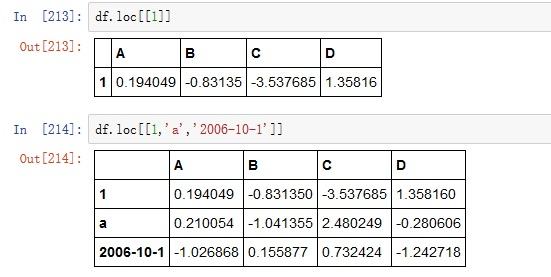ps：df.loc[]和df.loc返回结果的值相同，但数据结构有差异 方法2：.iloc['行号']or.iloc[['行号']]、.iloc[['行号1','行号2','行号n']] .iloc根据行号索引数据，行号是固定不变的，不受索引变化的影响，如果df的索引是默认值，则.loc和.iloc的用法没有区别，因为此时行号和行标签相同。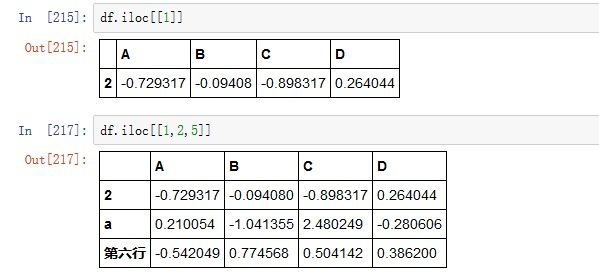可以看到df.loc和df.iloc读到的内容是不一样的，df.loc读取的是索引号为1的那一行，df.iloc读取的是第1行。 此外，.iloc可以通过切片的方式读取数据，所谓切片就是给出要读数据的首尾位置，然后读取首尾中间这“一片”数据（个人理解，可能理解的不对或比较片面，对此有疑惑的朋友请自行查阅相关资料）比如我们要读取第1行到第4行的数据，利用切片的方法就是df.iloc[1:5]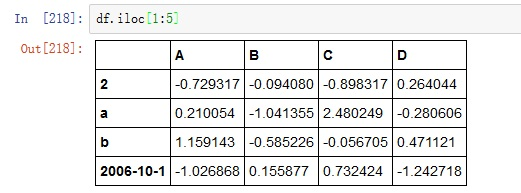如果从第0行开始读，则首位置可以省略，如果从某一行读到末尾，则尾位置可以省略。 df.iloc[:5]，读取第0行到第4行的数据； df.iloc[8:]，读取第8行后所有数据，包括第8行； df.iloc[3,6]，读取第3行到第6行的数据，包括第3行但不包括第6行。 方法3：.ix 根据其他网友的说法，.ix是.loc和.iloc的综合版，既可以通过行标签检索也可以根据行号检索。通过实验发现这个说法并不完成正确。以上面的df为例：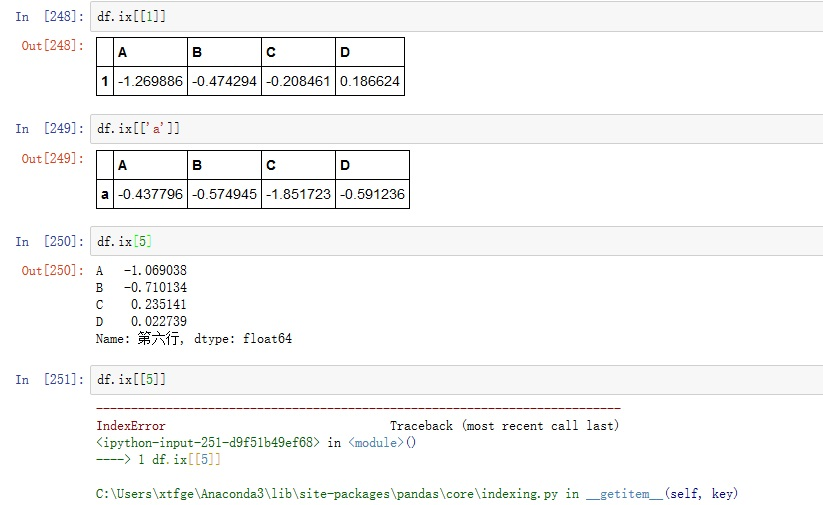因为df.ix[]可以认为是要读第1行，也可以认为要读索引为1的那一行，此时就产生了冲突，可以看到此时pandas认为df.ix[]读的是索引为1的那一行，如果用索引外的标签检索就会报错，而df.ix被认为是第1行，此时df.ix和df.ix[]读到的不是同一行数据。 如果我们将df的索引改为['a', 'b', 'c', 'd', 'e', 'f']再进行上面的操作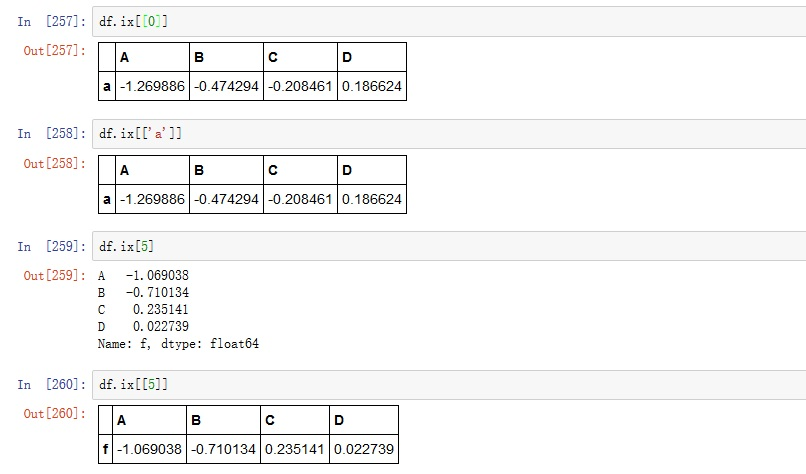此时df.ix和df.ix[]读到的是同一行数据。 通过以上实验，loc，iloc，ix的区别如下： loc通过索引标签读取数据； iloc通过行号读取数据； ix既可以通过行号读取数据，也可以通过索引标签读取数据，但是当索引为数字且不从0开始时，有两种情况： （1）每次读一行：通过索引标签读取和通过行号读取有不同的写法.ix[['列标签']],.ix[行号]。 （2）读取多行：此时只能通过索引标签，不能利用行号。 方法4：at、iat at、iat这里就不做介绍了，因为上面的方法完全够用了，感兴趣的话可以看官方文档。 .loc,.iloc完全可以满足DataFrame的读取操作，所以ix,at,iat并不推荐使用。
2.3 按单元格读取
方法1：df[col][row] 读取一个单元格的数据时推荐使用，也可以写成df.col[row]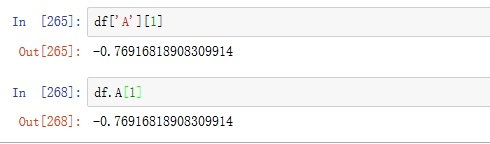方法2：.loc (1)读取一个单元格：df.loc[row][col]或df.loc[row,col] (2)读取一行多列：
df.loc[row][[col1,col2]]df.loc[1,[col1,col2]]df.loc[row][firstCol:endCol]df.loc[row,firstCol:endCol]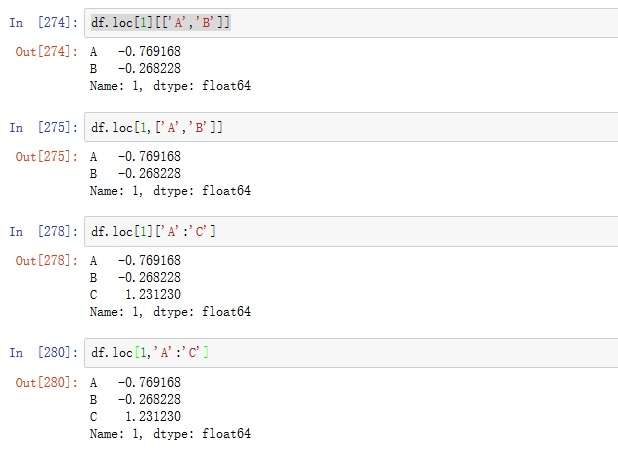(3)读取多行一列：df.loc[[row1,row2]][col]df.loc[[row1,row2]].coldf.loc[[row1,row2],col]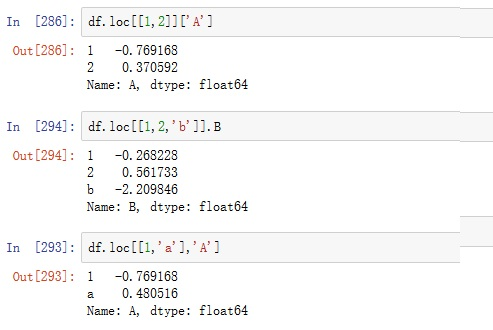ps :在这里行号不能用切片。 (4)读取多行多列df.loc[[row1,row2],[col1,col2]]df.loc[[row1,row2]][[col1,col2]]df.loc[[row1,row3],firstCol:endCol]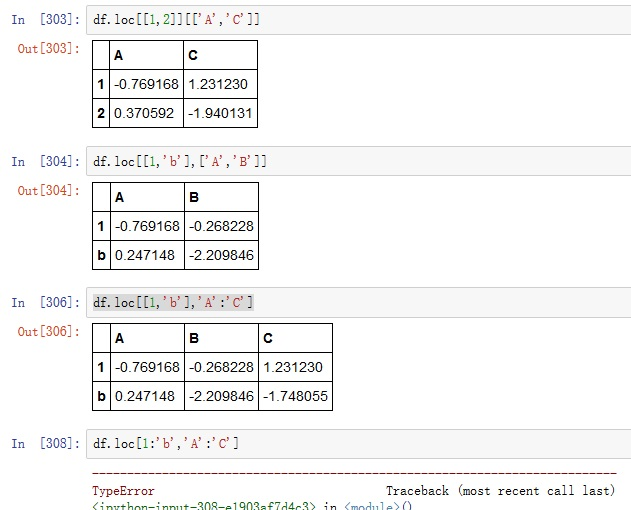ps：这里行号同样不能用切片，说明loc不支持行号切片索引。 方法3：.iloc (1)读取一个单元格df.iloc[rowNo].coldf.iloc[rowNo][col]df.iloc[rowNo,colNo] ps：df.iloc[rowNo,col]不支持。 (2)读取一行多列df.iloc[rowNo,firestColNo,endColNo]df.iloc[rowNo][[col1,col2]]df.iloc[rowNo][firesCol:endCol]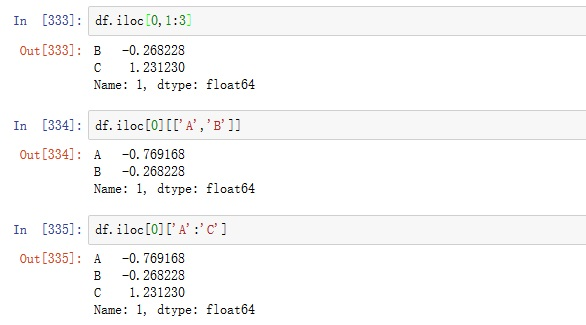ps：df.iloc[rowNo,[col1,col2]], df.iloc[rowNo,firstColNo:endColNo]均不支持。 (3)读取多行一列df.iloc[[rowNo1,rowNo2],colNo]df.iloc[firstRowNo:endRowNo,colNo]df.iloc[[rowNo1,rowNo2]][col]df.iloc[firstRowNo,endRowNo][col]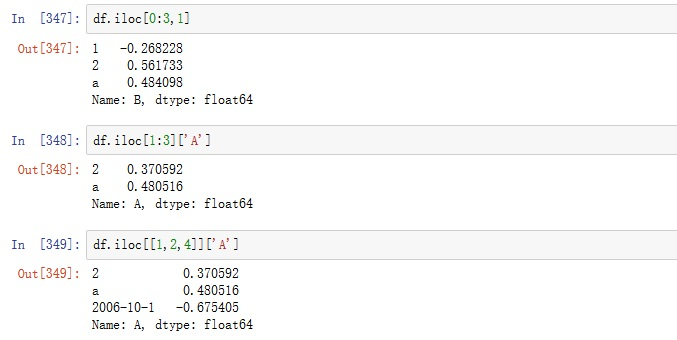(4)读取多行多列df.iloc[firstRowNo:endRowNo,firstColNo:endColNo]df.iloc[[RowNo1,RowNo2],[ColNo1,ColNo2]]df.iloc[firstRowNo:endRowNo][[col1,col2]]方法4：.ix 累的不行了，这里就直接写多行多列的读取吧。df.ix[firstRow/firstRowNo:endRow/endRowNo,firstCol/firstColNo:endCol/endColNo]df.ix[[row1/rowNo1,row2,rowNo2],[col1/colNo1,col2,colNo2]]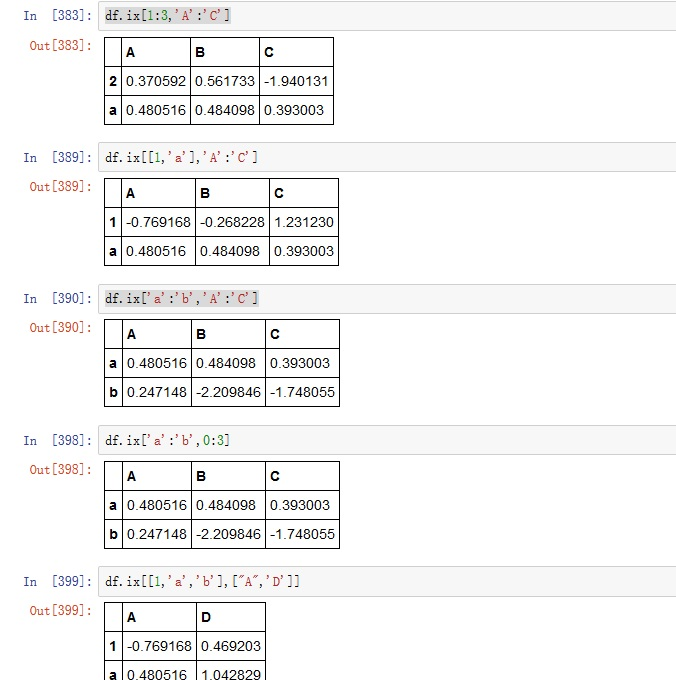方法5： at,iat
3 写DataFrame/DataFrame赋值
3.1 按列赋值
df.col=colList/colValuedf[col]=colList/colValue eg. df.A=[1,2,3,4,5,6],df['A']=0 ps1：如果用一个列表或数组赋值，其长度必须和df的行数相同。
3.2 按行赋值
df.loc[row]=rowListdf.loc[row]=rowValue
3.3 给多行多列赋值
df.loc[[row1,row2],[col1,col2]]=value/valueListdf.iloc[[rowNo1,rowNo2],[colNo1,colNo2]]=value/valueListdf.iloc[[rowNo1,rowNo2]][[col1,col2]]=value/valueListdf.ix[firstRow:endRow,firstCol:endCol]=value/valueList
ps：DataFrame的读写操作是多变的，这里也仅仅列出了几种常用的方法，熟练一种方式即可。
4 DataFrame的插入
以下面DataFrame为例展开说明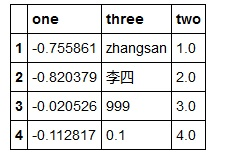4.1在任意位置插入
插入一列
insert(ioc,column,value)
ioc:要插入的位置
colunm:列名
value:值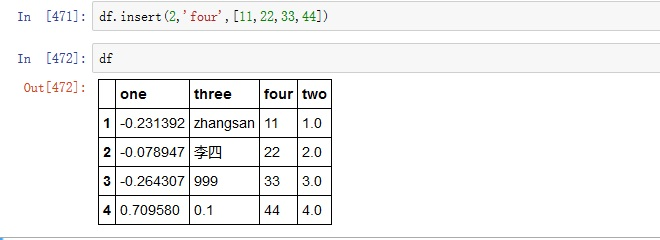插入一行
row={'one':111,'two':222,'three':333}
df.loc=row or
df.iloc=row or
df.ix=row or

4.2在末尾插入
如果插入一行或一列，用上面的方法把插入位置改为末尾即可，下面给出插入多行多列的方法。
pandas.concat(objs, axis=0, join_axes=None, ignore_index=False)
objs:合并对象
axis:合并方式，默认0表示按列合并，1表示按行合并
ignore_index:是否忽略索引

有df1和df2定义如下：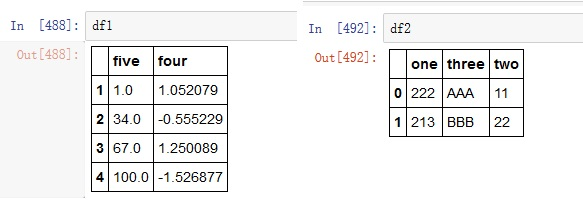按行合并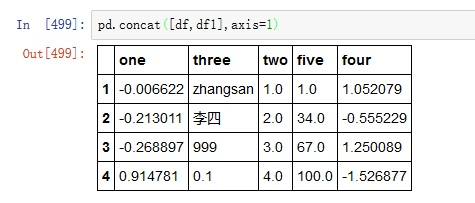按列合并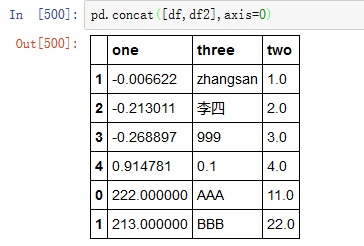利用append函数可以完成相同的操作: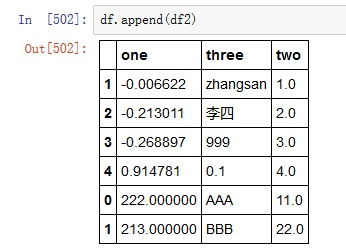5 DataFrame的删除操作
drop(labels, axis=0, level=None, inplace=False)
lables：要删除数据的标签
axis：0表示删除行，1表示删除列，默认0
inplace:是否在当前df中执行此操作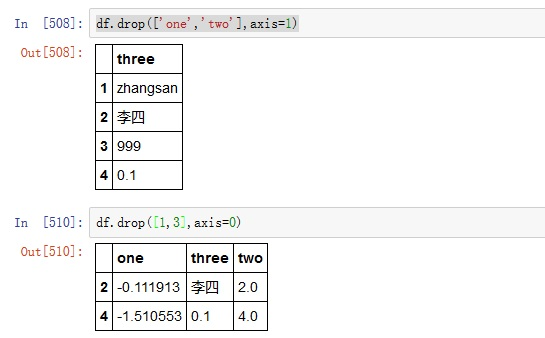后记：关于pandas的基本操作就写到这里了，关于以上内容如果有什么问题可以通过邮件联系博主，水平有限，请大家批评指正。 后面如果有机会，我会写关于DataFrame更进一步的操作，我也不知道会不会写，能写多少，先附上地址pandas进阶：DataFrame高级操作
展开全文DataFrame python
• (给Python开发者加星标，提升Python技能)作者：Jay Alammar，编译：极客猴如果读者们计划学习数据分析、机器学习、或者用 Python 做数据科学的研究，你会经常接触到 Pandas 库。Pandas 是一个开源、能用于数据操作和...pandas获取行号
• 方法一： 直接del DF[‘column-name’] 方法二： 用法：DataFrame.drop(labels=None,axis=0, index=None, columns=None, ...axis 默认为0，指删除行，因此删除columns时要指定axis=1； index 直接指定要删除的行 co...
• When deleting a column in a DataFrame I use: 在删除DataFrame中的列时，我使用： del df['column_name'] And thipython dataframe
• ## Pandas

2019-07-03 19:56:15
pandas有助于填补这一空白，使您能够在Python中执行整个数据分析工作流程，而无需切换到更像域的特定语言，如R. pandas是一个Python包，提供快速，灵活和富有表现力的数据结构 创建对象 导入包： import numpy as ...python 数据分析
• Pandas提供了duplicated、Index.duplicated、drop_duplicates函数来标记及删除重复记录 duplicated函数用于标记Series中的值、DataFrame中的记录行是否是重复，重复为True，不重复为False pandas.DataFrame....
• ## pandas

2018-01-31 15:10:19
pandas是基于numpy的一种工具，该工具是为了解决数据分析任务而创建的。pandas 纳入了大量库和一些标准的数据模型，提供了高效地操作大型数据集所需的工具。pandas提供了大量能使我们快速便捷地处理数据的函数和方法...Python
• 05_Pandas删除，替换并提取其中的缺失值NaN 例如，当使用pandas读取csv文件时，如果元素为空，则将其视为缺失值NaN（非数字）。 使用dropna（）方法删除缺失值，使用fillna（）方法用其他值替换（填充）缺失值。 ...python 机器学习
• Pandas提供了duplicated、Index.duplicated、drop_duplicates函数来标记及删除重复记录 duplicated函数用于标记Series中的值、DataFrame中的记录行是否是重复，重复为True，不重复为False pandas.DataFrame....
• 目录 Pandas 简介 下载 Pandas ...访问和删除 Pandas Series 中的元素 对 Pandas Series 执行算术运算 创建 Pandas DataFrame 使用Pandas Series 字典创建 DataFrame 使用列表（数组）字典创建 Dat...
• inplace=False，默认该删除操作不改变原数据，而是返回一个执行删除操作后的新dataframe； inplace=True，则会直接在原数据上进行删除操作，删除后就回不来了。 例子： >>>df = pd.DataFrame(np.arange(12).reshape......